#### Squeezing parts

Another example of sensorless manipulation will now be described, which was developed by Goldberg and Mason in [394,395,396]; see also . A Java implementation of the algorithm appears in . Suppose that convex, polygonal parts arrive individually along a conveyor belt in a factory. They are to be used in an assembly operation and need to be placed into a given orientation. Figure 12.50 shows a top view of a parallel-jaw gripper. The robot can perform a squeeze operation by bringing the jaws together. Figure 12.50a shows the part before squeezing, and Figure 12.50b shows it afterward. A simple model is assumed for the mechanics. The jaws move at constant velocity toward each other, and it is assumed that they move slowly enough so that dynamics can be neglected. To help slide the part into place, one of the jaws may be considered as a frictionless contact (this is a real device; see ). The robot can perform a squeeze operation at any orientation in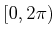(actually, only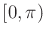is needed due to symmetry). Let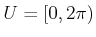denote the set of all squeezing actions. Each squeezing action terminates on its own after the part can be squeezed no further (without crushing the part).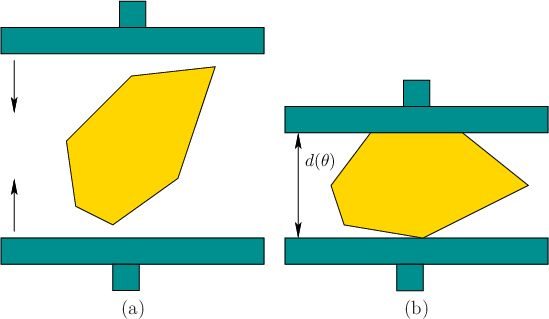The planning problem can be modeled as a game against nature. The initial orientation,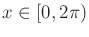, of the part is chosen by nature and is unknown. The state space is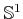. For a given part, the task is to design a sequence,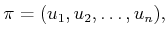(12.35)

of squeeze operations that leads to a known orientation for the part, regardless of its initial state. Note that there is no specific requirement on the final state. Aftermotion commands have terminated, the history I-state is the sequence(12.36)

of squeezes applied so far. The nondeterministic I-space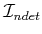will now be used. The requirement can be stated as obtaining a singleton, nondeterministic I-state (includes only one possible orientation). If the part has symmetries, then the task is instead to determine a single symmetry class (which includes only a finite number of orientations)

Consider how a part in an unknown orientation behaves. Due to rotational symmetry, it will be convenient to describe the effect of a squeeze operation based on the relative angle between the part and the robot. Therefore, let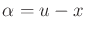, assuming arithmetic modulo. Initially,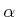may assume any value in. It turns out that after one squeeze,is always forced into one of a finite number of values. This can be explained by representing the diameter function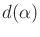, which indicates the maximum thickness that can be obtained by taking a slice of the part at orientation. Figure 12.51 shows the slice for a rectangle. The local minima of the distance function indicate orientations at which the part will stabilize as shown in Figure 12.50b. As the part changes its orientation during the squeeze operation, thevalue changes in a way that gradually decreases. Thus,can be divided into regions of attraction, as shown in Figure 12.52. These behave much like the funnels in Section 8.5.1.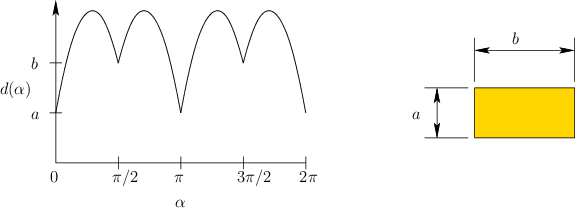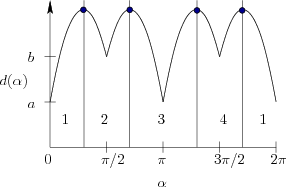The critical observation to solve the problem without sensors is that with each squeeze the uncertainty can grow no worse, and is usually reduced. Assumeis fixed. For the state transition equation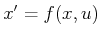, the same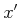will be produced for an interval of values for. Due to rotational symmetry, it is best to express this in terms of. Let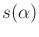denote relative orientation obtained after a squeeze. Sinceis a function ofand, this can be expressed as a squeeze function,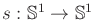, defined as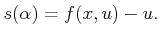(12.37)

The forward projection with respect to an interval,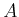, ofvalues can also be defined: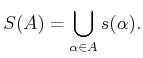(12.38)

Any interval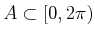can be interpreted as a nondeterministic I-state, based on the history of squeezes that have been performed. It is defined, however, with respect to relative orientations, instead of the original states. The algorithms discussed in Section 12.1.2 can be applied to. A backward search algorithm is given in  that starts with a singleton, nondeterministic I-state. The planning proceeds by performing a backward search on. In each iteration, the interval,, of possible relative orientations increases until eventually all ofis reached (or the period of symmetry, if symmetries exist).

The algorithm is greedy in the sense that it attempts to forceto be as large as possible in every step. Note from Figure 12.52 that the regions of attraction are maximal at the minima of the diameter function. Therefore, only the minima values are worth considering as choices for. Let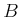denote the preimage of the function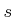. In the first step, the algorithm finds thefor which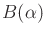is largest (in terms of length in). Letdenote this relative orientation, and let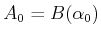. For each subsequent iteration, let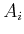denote the largest interval inthat satisfies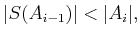(12.39)

in which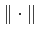denotes interval length. This implies that there exists a squeeze operation for which any relative orientation in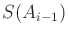can be forced intoby a single squeeze. This iteration is repeated, generating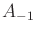,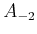, and so on, until the condition in (12.39) can no longer be satisfied. It was shown in  that for any polygonal part, theintervals increase until all of(or the period of symmetry) is obtained.

Suppose that the sequence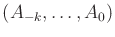has been computed. This must be transformed into a plan that is expressed in terms of a fixed coordinate frame for the robot. The-step action sequence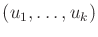is recovered from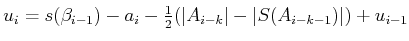(12.40)

and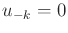. Each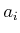in (12.40) is the left endpoint of. There is some freedom of choice in the alignment, and the third term in (12.40) selects actions in the middle to improve robustness with respect to orientation errors. By exploiting a proof in  that no more than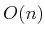squeeze operations are needed for a part withedges, the complete algorithm runs in time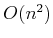.

Example 12..9 (Squeezing a Rectangle)   Figure 12.53 shows a simple example of a plan that requires two squeezes to orient the rectangular part when placed in any initial orientation. Four different executions of the plan are shown, one in each column. After the first squeeze, the part orientation is a multiple of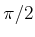. After the second squeeze, the orientation is known. Even though the execution looks different every time, no feedback is necessary because the I-state contains no sensor information.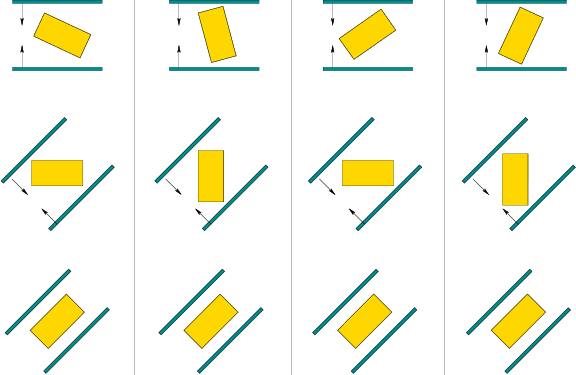Steven M LaValle 2020-08-14